# Search by Topic

#### Resources tagged with Interactivities similar to Taking Chances:

Filter by: Content type:
Age range:
Challenge level:

### There are 106 results

Broad Topics > Information and Communications Technology > Interactivities### Which Spinners?

##### Age 14 to 18 Challenge Level:

Can you work out which spinners were used to generate the frequency charts?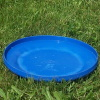### Flippin' Discs

##### Age 11 to 14 Challenge Level:

Discs are flipped in the air. You win if all the faces show the same colour. What is the probability of winning?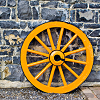### Interactive Spinners

##### Age 11 to 14 Challenge Level:

This interactivity invites you to make conjectures and explore probabilities of outcomes related to two independent events.### Two's Company

##### Age 11 to 14 Challenge Level:

Seven balls are shaken. You win if the two blue balls end up touching. What is the probability of winning?##### Age 14 to 16 Challenge Level:

A counter is placed in the bottom right hand corner of a grid. You toss a coin and move the star according to the following rules: ... What is the probability that you end up in the top left-hand. . . .### Cosy Corner

##### Age 11 to 14 Challenge Level:

Six balls are shaken. You win if at least one red ball ends in a corner. What is the probability of winning?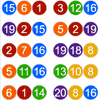### Lottery Simulator

##### Age 7 to 16 Challenge Level:

Use this animation to experiment with lotteries. Choose how many balls to match, how many are in the carousel, and how many draws to make at once.### Red or Black Spinner

##### Age 3 to 16 Challenge Level:

A simple spinner that is equally likely to land on Red or Black. Useful if tossing a coin, dropping it, and rummaging about on the floor have lost their appeal. Needs a modern browser; if IE then at. . . .### Excel Interactive Resource: Fraction Multiplication

##### Age 11 to 16 Challenge Level:

Use Excel to explore multiplication of fractions.### Semi-regular Tessellations

##### Age 11 to 16 Challenge Level:

Semi-regular tessellations combine two or more different regular polygons to fill the plane. Can you find all the semi-regular tessellations?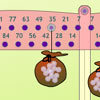### Balancing 2

##### Age 11 to 14 Challenge Level:

Meg and Mo still need to hang their marbles so that they balance, but this time the constraints are different. Use the interactivity to experiment and find out what they need to do.### Stars

##### Age 11 to 14 Challenge Level:

Can you find a relationship between the number of dots on the circle and the number of steps that will ensure that all points are hit?### Treasure Hunt

##### Age 7 to 14 Challenge Level:

Can you find a reliable strategy for choosing coordinates that will locate the treasure in the minimum number of guesses?### Isosceles Triangles

##### Age 11 to 14 Challenge Level:

Draw some isosceles triangles with an area of $9$cm$^2$ and a vertex at (20,20). If all the vertices must have whole number coordinates, how many is it possible to draw?### Square It

##### Age 11 to 16 Challenge Level:

Players take it in turns to choose a dot on the grid. The winner is the first to have four dots that can be joined to form a square.### Square Coordinates

##### Age 11 to 14 Challenge Level:

A tilted square is a square with no horizontal sides. Can you devise a general instruction for the construction of a square when you are given just one of its sides?### Right Angles

##### Age 11 to 14 Challenge Level:

Can you make a right-angled triangle on this peg-board by joining up three points round the edge?### More Number Pyramids

##### Age 11 to 14 Challenge Level:

When number pyramids have a sequence on the bottom layer, some interesting patterns emerge...### Number Pyramids

##### Age 11 to 14 Challenge Level:

Try entering different sets of numbers in the number pyramids. How does the total at the top change?### Excel Interactive Resource: Interactive Division

##### Age 11 to 16 Challenge Level:

Use an Excel to investigate division. Explore the relationships between the process elements using an interactive spreadsheet.### Attractive Tablecloths

##### Age 14 to 16 Challenge Level:

Charlie likes tablecloths that use as many colours as possible, but insists that his tablecloths have some symmetry. Can you work out how many colours he needs for different tablecloth designs?### Drips

##### Age 7 to 14 Challenge Level:

An animation that helps you understand the game of Nim.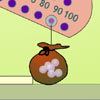### Balancing 1

##### Age 11 to 14 Challenge Level:

Meg and Mo need to hang their marbles so that they balance. Use the interactivity to experiment and find out what they need to do.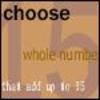### Fifteen

##### Age 7 to 14 Challenge Level:

Can you spot the similarities between this game and other games you know? The aim is to choose 3 numbers that total 15.### Excel Interactive Resource: Equivalent Fraction Bars

##### Age 11 to 16 Challenge Level:

A simple file for the Interactive whiteboard or PC screen, demonstrating equivalent fractions.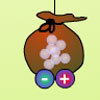### Balancing 3

##### Age 11 to 14 Challenge Level:

Mo has left, but Meg is still experimenting. Use the interactivity to help you find out how she can alter her pouch of marbles and still keep the two pouches balanced.### Excel Interactive Resource: Fraction Addition & Fraction Subtraction

##### Age 11 to 16 Challenge Level:

Use Excel to practise adding and subtracting fractions.### Substitution Cipher

##### Age 11 to 14 Challenge Level:

Find the frequency distribution for ordinary English, and use it to help you crack the code.### Excel Interactive Resource: Multiples Chain

##### Age 11 to 16 Challenge Level:

Use an interactive Excel spreadsheet to investigate factors and multiples.### Estimating Angles

##### Age 7 to 14 Challenge Level:

How good are you at estimating angles?### Excel Interactive Resource: Number Grid Functions

##### Age 11 to 16 Challenge Level:

Use Excel to investigate the effect of translations around a number grid.### Excel Interactive Resource: the up and Down Game

##### Age 11 to 16 Challenge Level:

Use an interactive Excel spreadsheet to explore number in this exciting game!### Percentages for Key Stage 4

##### Age 14 to 16 Challenge Level:

A group of interactive resources to support work on percentages Key Stage 4.### Icosian Game

##### Age 11 to 14 Challenge Level:

This problem is about investigating whether it is possible to start at one vertex of a platonic solid and visit every other vertex once only returning to the vertex you started at.### Overlap

##### Age 11 to 14 Challenge Level:

A red square and a blue square overlap so that the corner of the red square rests on the centre of the blue square. Show that, whatever the orientation of the red square, it covers a quarter of the. . . .### Proof Sorter - Quadratic Equation

##### Age 14 to 18 Challenge Level:

This is an interactivity in which you have to sort the steps in the completion of the square into the correct order to prove the formula for the solutions of quadratic equations.### Have You Got It?

##### Age 11 to 14 Challenge Level:

Can you explain the strategy for winning this game with any target?### Tilted Squares

##### Age 11 to 14 Challenge Level:

It's easy to work out the areas of most squares that we meet, but what if they were tilted?### Bow Tie

##### Age 11 to 14 Challenge Level:

Show how this pentagonal tile can be used to tile the plane and describe the transformations which map this pentagon to its images in the tiling.### Rolling Around

##### Age 11 to 14 Challenge Level:

A circle rolls around the outside edge of a square so that its circumference always touches the edge of the square. Can you describe the locus of the centre of the circle?### Rollin' Rollin' Rollin'

##### Age 11 to 14 Challenge Level:

Two circles of equal radius touch at P. One circle is fixed whilst the other moves, rolling without slipping, all the way round. How many times does the moving coin revolve before returning to P?### Guesswork

##### Age 14 to 16 Challenge Level:

Ask a friend to choose a number between 1 and 63. By identifying which of the six cards contains the number they are thinking of it is easy to tell them what the number is.### Konigsberg Plus

##### Age 11 to 14 Challenge Level:

Euler discussed whether or not it was possible to stroll around Koenigsberg crossing each of its seven bridges exactly once. Experiment with different numbers of islands and bridges.### Partitioning Revisited

##### Age 11 to 14 Challenge Level:

We can show that (x + 1)² = x² + 2x + 1 by considering the area of an (x + 1) by (x + 1) square. Show in a similar way that (x + 2)² = x² + 4x + 4### Jam

##### Age 14 to 16 Challenge Level:

To avoid losing think of another very well known game where the patterns of play are similar.### Sliding Puzzle

##### Age 11 to 16 Challenge Level:

The aim of the game is to slide the green square from the top right hand corner to the bottom left hand corner in the least number of moves.### Mixed up Mixture

##### Age 14 to 16 Challenge Level:

Can you fill in the mixed up numbers in this dilution calculation?### Changing Places

##### Age 14 to 16 Challenge Level:

Place a red counter in the top left corner of a 4x4 array, which is covered by 14 other smaller counters, leaving a gap in the bottom right hand corner (HOME). What is the smallest number of moves. . . .### Picturing Triangular Numbers

##### Age 11 to 14 Challenge Level:

Triangular numbers can be represented by a triangular array of squares. What do you notice about the sum of identical triangle numbers?### Investigating the Dilution Series

##### Age 14 to 16 Challenge Level:

Which dilutions can you make using only 10ml pipettes?